# H2O Binomial Scorer

Hi
I need to understand how does Binomial Scorere woks.
If you look to the P(section.type=emergency) the value is higher than the one on the other column. However, the prediction column contains the value “elective”.

# H2O Binomial Scorer

Using the regular scorer is actually referring to the two columns- the actual class label and the prediction labels. However, Binomial Scorer asks to provide the actually column and the probability column of the target class.
The confusion matric of the Binomial Scorers is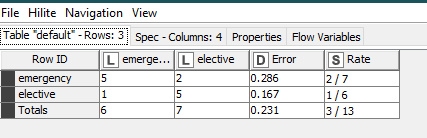While for regular Scorer is :So what is going here?

Best
Malik

Could you provide us with an example? Otherwise it is hard to tell what might be going on here.

OK
Part of the Knime Workflow: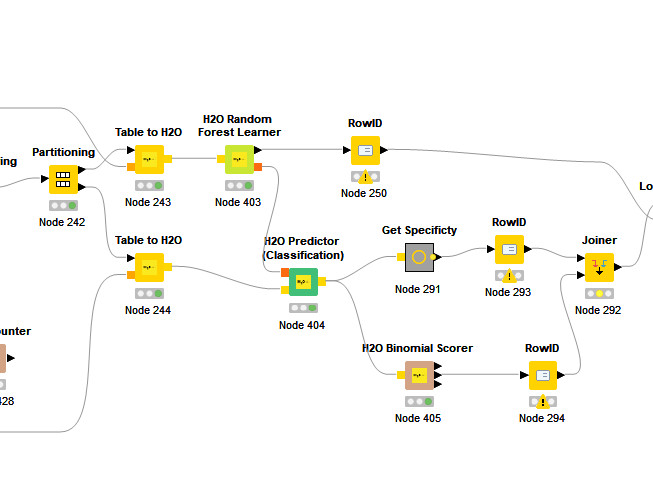Input Table -

Output of H2O Predictor (Classification)

Configure of H2O Binomial Scorer-Output of H2O Binomial Scorer- Confusion Matrix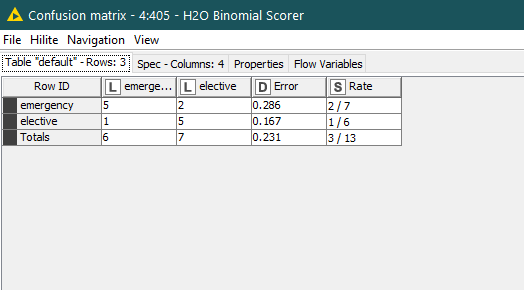If you could provide the workflow with the data it might be easier to judge what is going on. From what I see in the screenshots there seems to be a discrepancy between the score and the predicted output. Seems the H2O scorer is relying on the score and gives the case as emergency while the prediction is elective.

Besides a bug the thing I could think of is some sort of threshold that has to be passed in order to make a prediction. If there were some assigned costs for misclassification or something.

Might be a case for the H2O people. Anyway it would be good to have an example that can be reproduced.

1 Like

Hi @malik and @mlauber71,

H2O optimizes the threshold that they will use for the prediction during the model learning. If you take a look at the output of the H2O Random Forest Learner node, you will find a flow variable called “Threshold”. This is the optimized threshold that will be used by the H2O Binomial Scorer. If you want to use a different threshold, you need to use either the Scorer node for 0.5 as threshold, or a Rule Engine node for a custom threshold.

I hope this clarifies it.

Regards,
Simon

3 Likes

You might doenload the workflow from

Input file \:
data_clean_malik_26_9_209.xlsx (158.8 KB)

So no way that we do that with the H2O Binomial Scorer ?

Malik

You could use The Rule Engine and define the Threshold yourself. As @SimonS explained H2O.ai uses an optimized threshold so there is not a simple split at a score of 0.5.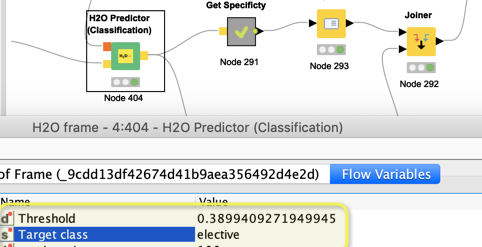The best Threshold/prediction very much depends on your business model and what you want to do with the results.

You could read more in these entries, especially about the ROC curve.

Models for 0/1 or Yes/No Targets

Understand metrics like AUC and Gini (and use H2O.ai)

1 Like

It should more simple to solve this issue. I notice that node H2O Binomial Scorer allow one to choose just INTEGER column as the Predicted column (target class) - So in my case i use the Actual column to be “section.type” and the target class is “elective” and the Prediction columns (target class) is the "P(section.type=elective)).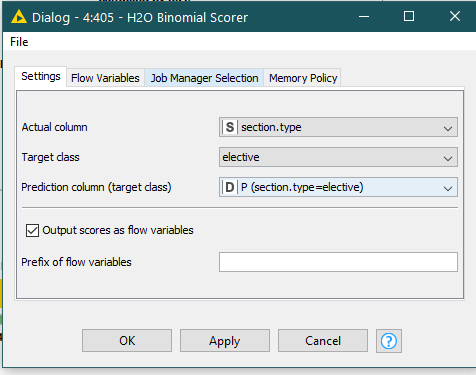Now examine the output of the H2O Binomial Scorer ->Confusion matrixthe results seems correct.
So i need just to calculate the specific from this table.
I don’t know why H2O Binomial Scorer calculate all different measurement and not the specificity!!!

This is my solution
provide the neg class name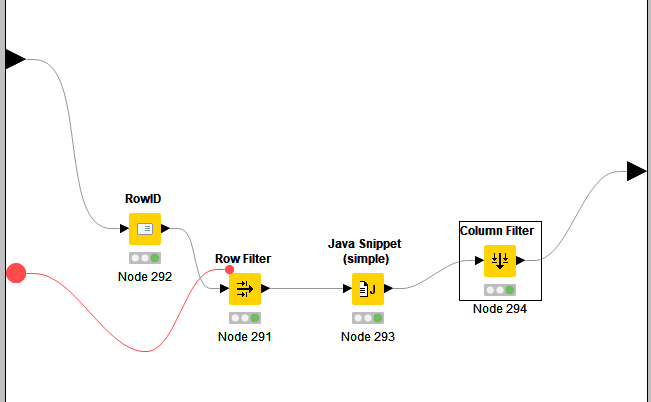in java snippet : return (1-\$Error\$);

Malik

2 Likes

This topic was automatically closed 182 days after the last reply. New replies are no longer allowed.